# k-server, part 1: online learning and online algorithms

The-server problem is a classical and very attractive instance of online decision making. The decisions to be made in this problem are simple: given a requested location in some finite metric space and a fleet of k servers currently sitting in this metric space, one has to choose which server to use to service the request (that is the server will move from its current location to the requested location). The problem is to minimize the total amount of movement of the servers on a stream of such requests. This formulation is both abstract enough that it can model many real-life problems (e.g., virtual memory management) yet concrete/simple enough that it seems that everything ought to be understood about it.

In the coming series of 5 blog posts I will explain the main ideas behind our-server preprint with Michael Cohen, James Lee, Yin Tat Lee, and Aleksander Madry. In this first post I will briefly put the problem in the broader context of online learning and online algorithms, which will be helpful as it suggests an approach based on the mirror descent algorithm. In the next post I will explain the general framework of mirror descent and how it can be applied to a problem such as k-server. The third post will show how to use this general framework to recover effortlessly the state of the art for weighted k-paging (i.e., when the underlying metric space is a weighted star). The fourth post will show how to extend the analysis to arbitrary trees. Finally in the fifth post we will discuss both classical embedding techniques to reduce the problem to the tree case, as well as our new dynamic embedding based on multiscale restarts on the classical technique. The content of the first three posts was essentially covered in my TCS+ seminar talk.

Online decision making: two probability free models

I will start by introducing a very general framework for online algorithms due to Borodin, Linial and Saks 92, called metrical task systems (MTS). At the same time I will recall the online learning framework, so that one can see the similarities/differences between the two settings. In fact this connection was already made at the end of the Nineties, see Blum and Burch 00. At the time it was natural to explore the power of multiplicative weights. With today’s perspective it is natural to explore the much more general mirror descent algorithm (we note that the closely related concept of regularization was already brought to bear for these problems in Abernethy, Bartlett, Buchbinder and Stanton 10, and Buchbinder, Chen and Naor 14).

Let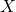be a set which we think of as either a state space (for online algorithms) or an action space (for online learning). Let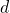be a metric on. Finally let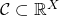be a set of possible cost functions (e.g., arbitrary bounded functions, or linear functions if, or convex functions, etc). Online decision making is about making decisions in an uncertain environment (the future is not known), and here we model this uncertain environment as an unknown sequence of cost functions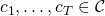. The interaction protocol can then be described as follows: For each, the player chooses (possibly randomly)based on past observations (to be made precise soon) and pays the cost, plus possibly a movement penalty:.

There are now two key differences between online algorithms and online learning: (i) the observation model, and (ii) the performance metric. In online learning one assumes that the costis unknown at the decision time, and is only revealed after the decision is made (in bandits an even weaker signal is revealed, namely only the paid cost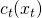). The type of applications one has in mind is say online recommendations, where a user’s preference is only revealed once some item has been recommended to her. On the other hand in online algorithms the costis known at decision time. In this context the cost corresponds to a request, and the player has to find a way to satisfy this request (in the language of MTS the cost represents a task, and one gets the option to update its state so as to complete this task more efficiently, i.e., at a lower cost). Now let us discuss the performance metric. In online learning one assumes that there is some “hidden” good action (it is hidden in the noise, i.e., a single cost function does not say much about which actions are good, but if one takes the whole sequence into account then there is some good action that hopefully emerges). Thus it is natural to consider the following regret notion:This regret notion does not make sense for online algorithms where one may have to keep changing states to satisfy the request sequence. There one must compare to the best offline strategy, in which case additive guarantees are not attainable and one resorts to a multiplicative guarantee, the so-called competitive ratio:(Note that in MTS one always assumes nonnegative cost functions so that the multicative guarantee makes sense.) The-server problem, introduced in Manasse, McGeoch and Sleator 90, corresponds to a metrical task system on the product state spaceequipped with the Earthmover distance inherited by, and with cost functionsKnown results

The online learning setting is by now fairly well-understood. We know that the optimal regret for a finite action setand bounded cost functions is(see e.g., Cesa-Bianchi, Freund, Haussler, Helmbold, Schapire and Warmuth 97). In fact we even know the optimal constant (for large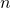and), and we have both algorithmic and information theoretic proofs of this result. Moreover we know how to leverage combinatorial information, e.g., whenand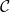is a set of linear functions. A unifying principle that was put forth in the last decade or so is the mirror descent strategy.

On the other hand the situation for MTS is much worse. The optimal guarantees for finite sets are not known: the worst case (over all metric spaces of size) competitive ratio is known to be(trivial coupon-collector style lower bound on the uniform metric) and(the latter bound is due to Fiat and Mendel 03). No information theoretic analysis is known, even for the uniform metric. With combinatorial structure the situation becomes even more disastrous. That’s where the-server problem comes in, as a combinatorial MTS striking the best balance between simplicity and importance. For-server it is conjectured that one could get a competitive ratio of(the coupon-collector lower bound on uniform metric gives here), while the best result prior to our work was(due to Bansal, Buchbinder, Naor and Madry 11), and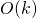if one insists for a bound independent of(due to Koutsoupias and Papadimitriou 95).

This entry was posted in Theoretical Computer Science. Bookmark the permalink.

## 4 Responses to "k-server, part 1: online learning and online algorithms"

••